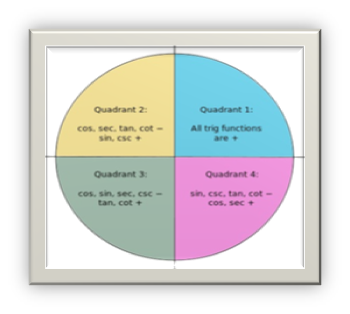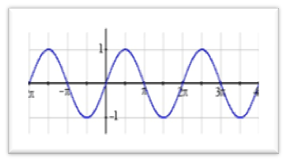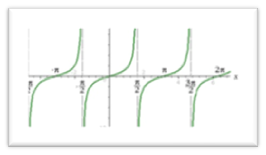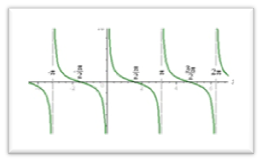Click to Chat

1800-1023-196

+91-120-4616500

CART 0

• 0

MY CART (5)

Use Coupon: CART20 and get 20% off on all online Study Material

ITEM
DETAILS
MRP
DISCOUNT
FINAL PRICE
Total Price: Rs.

There are no items in this cart.
Continue Shopping• Complete JEE Main/Advanced Course and Test Series
• OFFERED PRICE: Rs. 15,900
• View Details

```Revision Notes on Trigonometric Functions

The trigonometric ratios are the ratios between the two sides of a right-angles triangle with respect to an angle and hence they are real numbers.

The angle θ taken into consideration may be acute, obtuse or right angle.

Various trigonometry formulas related to the basic trigonometric ratios which must be remembered include:

1) sin2 θ + cos2 θ = 1

2) sec2 θ -tan2 θ = 1

3) cosec2 θ - cot2 θ = 1

4) sin θ cosec θ = tan θ cot θ = sec θ cos θ = 1

5) sin2 θ + cos2 θ = 1, so each of them is numerically less than 1.

6) |sin θ| ≤ 1 and |cos θ| ≤ 1

7) -1 <cosec θ<1 and -1 <sec θ<1

8) tan θ and cot θ may take any value.

The trigonometry ratios can be either positive or negative depending on the quadrant in which they lie. The figure given below illustrates the various trigonometric ratios and their signs in various quadrants:Range of f: X →Y is the set of all images f(x) which belongs to Y, i.e.

Range f = {f(x) ∈ Y: x ∈ X} ⊆ Y.

The domain and range of various trigonometry ratios are given below:

Trigonometric Function

Domain

Range

sin x

R

-1 ≤ sin x ≤1

cos x

R

-1 ≤ cos x ≤1

tan x

R – {(2n + 1)π/2, n ∈ I}

R

cosec x

R – {nπ, n ∈ I}

R – {x: -1 < x <1}

sec x

R – {(2n + 1)π/2, n ∈ I}

R – {x: -1 < x <1}

cot x

R – {nπ, n ∈ I}

R

Given below is the trigonometry table of the basic ratios for some frequently used angles. These formulas must be memorized as they are used in almost all questions:

Angle (x)

sin x

cos x

tan x

cosec x

sec x

cot x

0°

0

1

0

undefined

1

undefined

90° = π/2

1

0

undefined

1

undefined

0

180° = π

0

-1

0

undefined

-1

undefined

270° =3π/2

-1

0

undefined

-1

undefined

0

360° = 2π

0

1

0

undefined

1

undefined

Some other formulas of trigonometry which are useful in solving questions:

Angles

0°

30°

45°

60°

90°

sin

0

1/2

1/√2

√3/2

1

cos

1

√3/2

1/√2

1/2

0

tan

0

√3/2

1

√3

undefined

cosec

undefined

2

√2

2/√3

1

sec

1

2/√3

√2

2

undefined

cot

undefined

√3

1

1/√3

0

The values of various trigonometric ratios vary in different quadrants. The variation is listed below quadrant wise:

1. sine- increases from 0 to 1

2. cosine- decreases from 1 to 0

3. tangent- increases from 0 to ∞

4. cotangent- decreases from ∞ to 0

5. secant- increases from 1 to ∞

6. cosecant- decreases from ∞ to 1

1. sine- decreases from 1 to 0

2. cosine- decreases from 0 to -1

3. tangent- increases from -∞ to 0

4. cotangent- decreases from 0 to -∞

5. secant- increases from -∞ to -1

6. cosecant- increases from 1 to ∞

1. sine- decreases from 0 to -1

2. cosine- increases from -1 to 0

3. tangent- increases from 0 to ∞

4. cotangent- decreases from ∞ to 0

5. secant- decreases from -1 to -∞

6. cosecant- increases from -∞ to -1

1. sine- increases from -1 to 0

2. cosine- increases from 0 to 1

3. tangent- increases from -∞ to 0

4. cotangent- decreases from 0 to -∞

5. secant- decreases from ∞ to 1

6. cosecant- decreases from -1 to ∞

Sin and cos both have 2π as their period.

tan function has π as the period.

The reciprocal functions have the same period as that of the original functions.

If the period of f(x) is T then that of kf(ax+b) is T/mod (a), hence period is affected by coefficient of x only.

If f(x) has its period T and g(x) has its period M, then (af(x) + bg(x)) has its period < L.C.M. (T, M). Moreover if f(x) and g(x) are basic trigonometric functions then period of [af (x) + bg(x)] = L.C.M. (T, M).

If a constant is added, subtracted, multiplied or divided in a periodic function, its period is unaffected.

Graphs of some basic trigonometric functions:

1) sin x2) cos x3) tan x4) cot x5) sec x```### Course Features

• 728 Video Lectures
• Revision Notes
• Previous Year Papers
• Mind Map
• Study Planner
• NCERT Solutions
• Discussion Forum
• Test paper with Video Solution